Courses

# 28 Year NEET Questions: Dual Nature of Radiation and Matter- 2

## 30 Questions MCQ Test Physics 28 Years Past year papers for NEET/AIPMT Class 12 | 28 Year NEET Questions: Dual Nature of Radiation and Matter- 2

Description
This mock test of 28 Year NEET Questions: Dual Nature of Radiation and Matter- 2 for NEET helps you for every NEET entrance exam. This contains 30 Multiple Choice Questions for NEET 28 Year NEET Questions: Dual Nature of Radiation and Matter- 2 (mcq) to study with solutions a complete question bank. The solved questions answers in this 28 Year NEET Questions: Dual Nature of Radiation and Matter- 2 quiz give you a good mix of easy questions and tough questions. NEET students definitely take this 28 Year NEET Questions: Dual Nature of Radiation and Matter- 2 exercise for a better result in the exam. You can find other 28 Year NEET Questions: Dual Nature of Radiation and Matter- 2 extra questions, long questions & short questions for NEET on EduRev as well by searching above.
QUESTION: 1

### The energy of a photon of wavelength λ is 

Solution:

Energy of a photon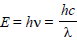QUESTION: 2

### The threshold frequency for photoelectric effecton sodium corresponds to a wavelength of5000 Å. Its work function is 

Solution:

W=hc/ λ
h=6.6×10-34J s
c=3×108m/s
λ =5000Å=5×10-7m
W=hc/ λ
={6.6×10-34×3×108}/(5×10-7)
=3.96×10-19J∼4x10-19J

QUESTION: 3

### The de -Broglie wave corresponding to a particle of mass m and velocity v has a wavelength associated with it 

Solution:

De-Broglie wavelength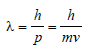QUESTION: 4

Energy levels A, B, C of a certain atom correspond to increasing values of energy i.e., EA < EB < EC. If λ1, λ2, λ3 are the wavelengths of radiation corresponding to the transitions C to B, B to A and C to A respectively, which of the following relation is correct? [1990, 2005]

Solution: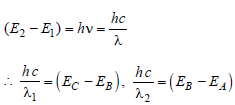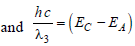Now,
(EC - EA) = (EC - EB ) + (EB - EA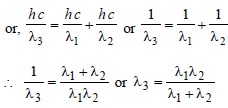)

QUESTION: 5

A radio transmitter operates at a freqency 880kHz and a power of 10 kW. The number ofphotons emitted per second is 

Solution:

No. of photons emitted per sec,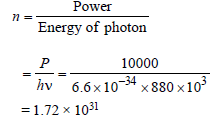QUESTION: 6

The momentum of a photon of an electromagneticradiation is 3.3 × 10–29 kgms–1. What is thefrequency of the associated waves ? [h = 6.6 × 10–34 Js; c = 3 × 108 ms–1)            

Solution:

p=3.3×10−29 kgms−1
λ=h/mv
c/v=h/p​
v=pc/h​
=(3.3×10−29×3×108​)/(6.6×10−34)
=1.5×1013Hz
So, the answer is option (D).

QUESTION: 7

Consider an electron in the nth orbit of ahydrogen atom in the Bohr model. Thecircumference of the orbit can be expressed interms of de-Broglie wavelength λ of that electronas 

Solution:

The circumference of an orbit in an atom in
terms of wavelength of wave associated with
electron is given by the relation,
circumference = nλ
where, n = 1, 2, 3, .......

QUESTION: 8

The wavelength of a 1 keV photon is 1.24 × 10–9 m. What is the frequency of 1 MeV photon ? 

Solution:

Here, hc/λ  = 103 eV and hv = 106 eV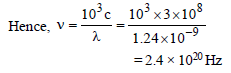QUESTION: 9

Photoelectric work function of a metal is 1eV.Light of wavelength λ�� = 3000 Å falls on it. Thephoto electrons come out with velocity 

Solution: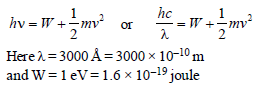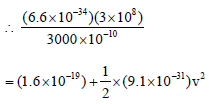Solving we get, v ≌ 106 m/s

QUESTION: 10

The cathode of a photoelectric cell is changedsuch that the work function changes from W1 toW2 (W2 > W1). If the current before and afterchanges are I1 and I2, all other conditionsremaining unchanged, then (assuming hν > W2)

Solution:

The work function has no effect on
photoelectric current so long as hv > W0.
The photoelectric current is proportional to
the intensity of incident light. Since there is
no change in the intensity of light, hence I1
= I2.

QUESTION: 11

An ionization chamber with parallel conductingplates as anode and cathode has 5 × 107 electronsand the same number of singly charged positiveions per cm3. The electrons are moving towards the anode with velocity 0.4 m/s. The currentdensity from anode to cathode is 4μA/m2. Thevelocity of positive ions moving towardscathode is     

Solution:

Current = Ie+ Ip
Ie and Ip are current due to electrons and
positively charged ions.
I = neAVd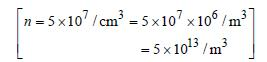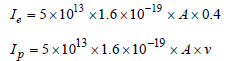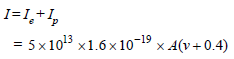Given, I / A = 4 x10-6 A/ m2

4x10-6 x A = 5 x10-6 x1.6 x A(v + 0.4)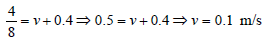QUESTION: 12

When light of wavelength 300 nm (nanometer) falls on a photoelectric emitter, photoelectronsare liberated. For another emitter, however, light of 600 nm wavelength is sufficient for creatingphotoemission. What is the ratio of the work functions of the two emitters?  

Solution:

Work function   ϕ=hc​/λ
⟹ ϕ12​ ​​=​λ2​​/λ1
Given :  λ1​=300 nm          λ2​=600 nm
∴  ​ϕ1/ ϕ2 ​​=600/300 ​=2

QUESTION: 13

Number of ejected photoelectron increases withincrease    

Solution:

Photoelectric current is directly proportional
to the intensity of incident light.

QUESTION: 14

Momentum of a photon of wavelength λ is

Solution:

According to de Brogie wave equation,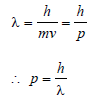QUESTION: 15

In photoelectric effect the work function of ametal is 3.5 eV. The emitted electrons can bestopped by applying a potential of –1.2 V. Then

Solution:

hv = W0 + Ek = 3.5 + 1.2 = 4.7 eV

QUESTION: 16

Doubly ionised helium atoms and hydrogen ions are accelerated from rest through the same potential drop. The ratio of the final velocities of the helium and the hydrogen ion is 

Solution:

Solution :- Ionised atoms = Helium (Given)

Ionised ions = Hydrogen (Given)

Potential difference = constant (Given)

Thus, mv² = eV, or

V = (2eV/m)1/2

The mass of helium ion is four times than that of hydrogen ion.

Therefore, the charge on helium ion is twice that of hydrogen ion.

vHe/vH = √2

Thus, The ratio of final velocities of helium and hydrogen is √2:1

QUESTION: 17

Gases begin to conduct electricity at low pressurebecause 

Solution:

The ionisation requires high energy
electrons.

QUESTION: 18

Kinetic energy of an electron, which isaccelerated in a potential difference of 100 V is

Solution:

Potential difference (V) = 100 V. The kinetic
energy of an electron = 1 eV = 1 × (1.6 × 10–19)
= 1.6 × 10–19 J. Therefore kinetic energy in
100 volts = (1.6 × 10–19) × 100 = 1.6 × 10–17J.
[Alt : K.E. = qV
= 1.6 × 10–19 × 100 J = 1.6 × 10–17]

QUESTION: 19

The wavelength associated with an electron,accelerated through a potential difference of 100V, is of the order of 

Solution:

Potential difference = 100 V
K.E. acquired by electron = e (100)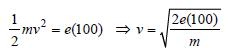According to de Broglie's concept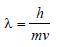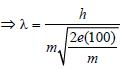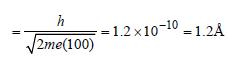QUESTION: 20

An electron of mass m and charge e is accelerated from rest through a potential difference of V volt in vacuum. Its final speed will be 

Solution:

Kinetic energy of electron accelerated
through a potential V= eV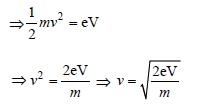QUESTION: 21

Which of the following statement is correct?   

Solution:

According to photoelectric effect, speed of
electron (kinetic energy) emitted depends
upon frequency of incident light while
number of photoelectrons emitted depends
upon intensity of incident light. Hence, as
the intensity of light increases, the
photocurrent increases. In a photo-cell, the
photocurrent has no relation with the applied
voltage.
Stopping potential is the (negative) potential
at which the current is just reduced to zero.
It is independent of intensity of light but
depends on the frequency of light similar to
K.E.

QUESTION: 22

Cosmic rays are 

Solution:

Cosmic rays have low wavelength and high
frequency hence these rays emit high energy

QUESTION: 23

The X-rays cannot be diffracted by means of anordinary grating because of         

Solution:

We know that the X-rays are of short
wavelength as compared to grating constant
of optical grating. As a result of this, it makes
difficult to observe X-rays diffraction with
ordinary grating.

QUESTION: 24

In a photo-emissive cell, with exciting wavelength λ, the fastest electron has speed v. If the exciting wavelength is changed to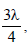the speed of the fastest emitted electron will be 

Solution: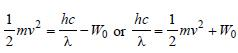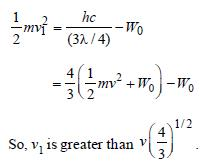QUESTION: 25

The 21 cm radio wave emitted by hydrogen ininterstellar space is due to the interaction called the hyperfine interaction in atomic hydrogen.The energy of the emitted wave is nearly       

Solution:

E=hc/λ​=6.6×10−34×3×108​/21×10−2 =0.94×10−24≈10−24J

QUESTION: 26

Light of wavelength 5000 Å falls on a sensitiveplate with photo-electric work function of 1.9 eV.The kinetic energy of the photo-electronsemitted will be 

Solution:

From photoelectric equation
KE=hc/λ​−W
(6.6×10−34×3×108/5000×10−10)−(1.9×1.6×10−19)
=0.58eV

QUESTION: 27

As the intensity of incident light increases        

Solution:

K.E. of electrons emitted depends upon the
frequency of incident rays rather than the
intensity. While number of photo electrons
emitted depends upon intensity of radiation.

QUESTION: 28

The photoelectric work function for a metalsurface is 4.125 eV. The cut off wavelength forthis surface is 

Solution:

Let λ0 be cut off wavelength.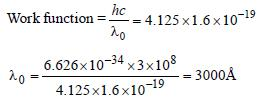QUESTION: 29

Einstein work on the photoelectric effect provided support for the equation          

Solution:

Einstein work on photoelectric effect
supports the equation E = hv.It is based on
quantum theory of light.

QUESTION: 30

Which of the following moving particles (movingwith same velocity) has largest wavelength ofmatter waves? 

Solution:

de-Broglie wavelengthFor same velocity,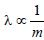Out of given particles, the mass of electron
is minimum, so the associated de-Broglie
wavelength is maximum for electron.